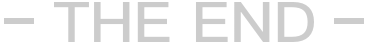### 找到include/common.func.php,打开这个文件，在这个文件的最后加入如下代码：

```function GetTopTypename(\$id)
{
global \$dsql;
\$row = \$dsql->GetOne("SELECT typename,topid FROM dede_arctype WHERE id= \$id");
if (\$row['topid'] == '0')
{
return \$row['typename'];
}
else
{
\$row1 = \$dsql->GetOne("SELECT typename FROM dede_arctype WHERE id= \$row[topid]");
return \$row1['typename'];
}
}//这里需要注意的是这个已经是到达了文件的末尾了下面千万不要出现换行。

```